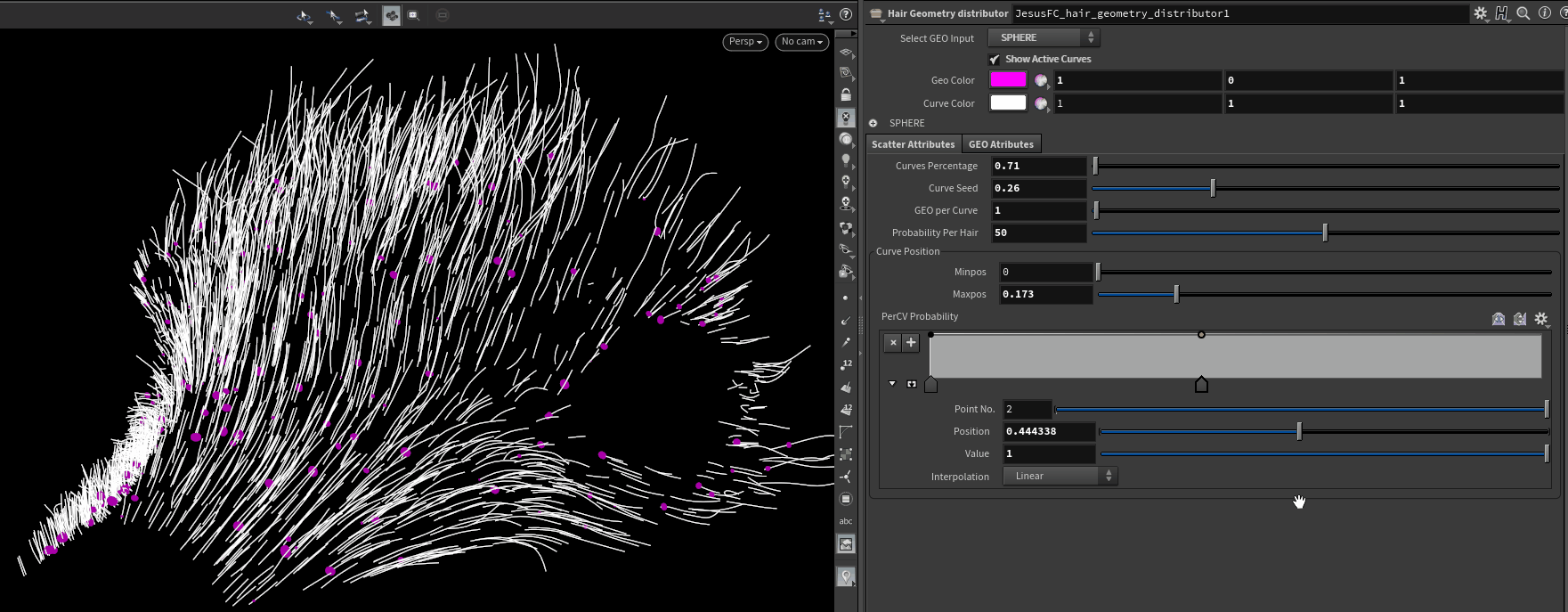August 19, 2021

## HAIR GEOMETRY DISTRIBUTOR## General Controls

Active Curve

Displays the percentage of curves to be used for the distribution.

Curve Color

Changed the color preview of the Active guide curves.

Geo Color

Changed the color preview of the distributed Geometries.

## Scatter Attributes

#### Curve Percentage

Controls the percentage of curves selected to spawn the geometries. Works from 0% – 100%.

#### Curve Seed

Changes the seed of the selected curves. This will have no effect at either 0% or 100%.

#### Geo Per Curve

Controls the amount of Geometries per curve.

#### Probability Per Curve

Adjusts the probability of a geometry to spawn on the curve. Works as a multiplier of the density.

#### Min Max Position

Controls the position of the Geometry on the curve based on the length. You can go higher than 1 to compress the Geometries on said point.

Defines the probability of a Geometry to appear on the curves based on a 0 to 1 ramp.

0 = root

1 = tip

## GEO ATTRIBUTES

#### Global Percentage

Controls the percentage of generated geometries as an absolute value on all the curves. Serves for preview purposes.

#### Location Seed

Controls the randomness of the global percentage selection.

#### Global Scale

Multiplies the scale of all the geometries being scattered on the geometry.

#### Per Axis Scale

Controls the scale per axis. Works as a multiplier.

#### Random Scale

Min = Defines the minimum scale value for the geometries to use.
Max = Defines the maximum scale values for the geometries to use.
Seed = Changes the sampled multiplier for all geos.

#### Scale Ramp Control

Defines the scale of a Geometry to appear on the curves based on a 0 to 1 ramp.

0 = root

1 = tip

#### Rotation Angle

Multiplies the rotation of all the geometries being scattered on the geometry.

#### Random Rotation

Randomly sets a rotation value for each geometry.

#### Rotate Ramp Control

Defines the rotation of a Geometry to appear on the curves based on a 0 to 1 ramp.

0 = root

1 = tip

#### Translate

Moves all the geometries on the defined axis.

#### Translate Ramp Control

Defines the translation of a Geometry to appear on the curves based on a 0 to 1 ramp.

0 = root

1 = tip

#### Simple Translation

Translates the geometries on on both directions of the defined axis using the curve as the center.. If axis is X, translates the geometries on the curve by -X and +X .

Seed = Samples a different value per geometry.

#### Random Translation

Translates the geometries on randomly in all directions.
Min = Defines the minimum translation per geometry. Max = Defines the maximum translation per geometry. Seed = Changes the sampled multiplier for all geometries.

#### Translate Random Ramp Control

Defines the random translation of a Geometry to appear on the curves based on a 0 to 1 ramp.

0 = root

1 = tip